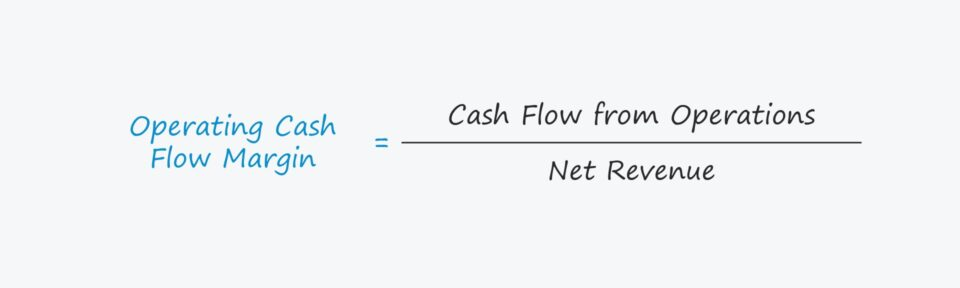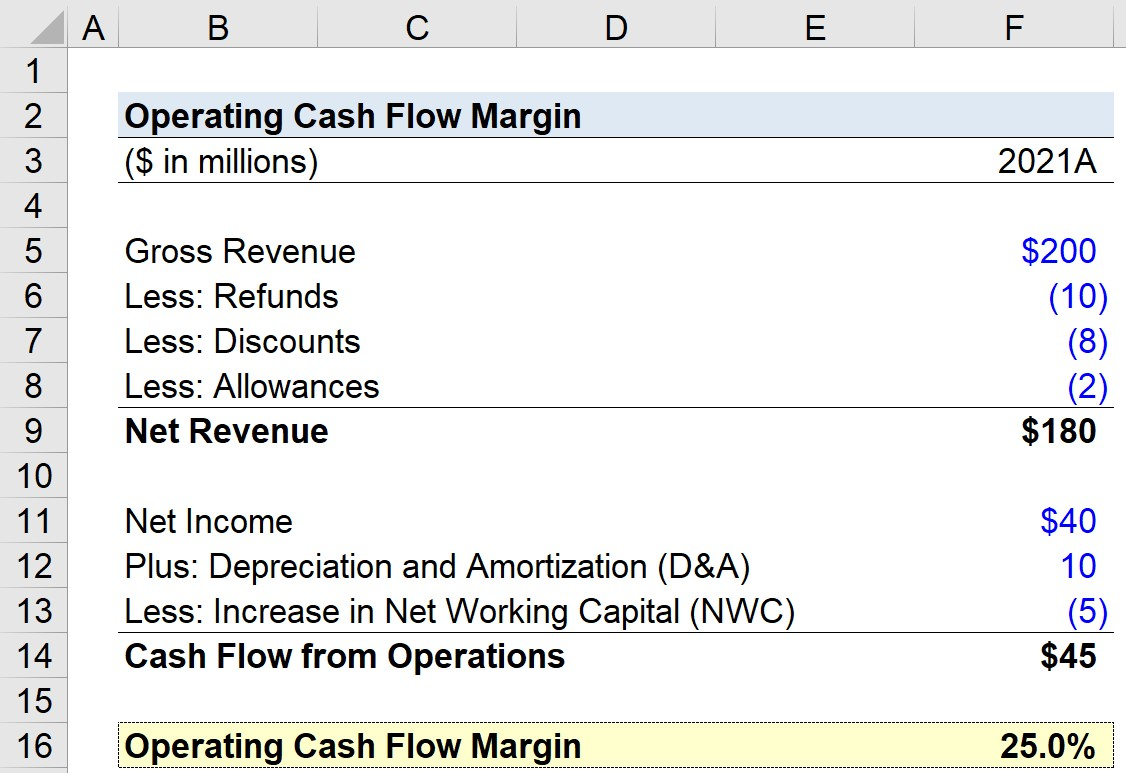Welcome to Wall Street Prep! Use code at checkout for 15% off.# Operating Cash Flow Margin

Guide to Understanding the Operating Cash Flow Margin## How to Calculate Operating Cash Flow Margin?

The operating cash flow margin is a profitability ratio that compares a company’s operating cash flow to its net revenue over a specified period.

• Operating Cash Flow (OCF) → OCF represents the net cash generated from a company’s day-to-day operations across a given time span.
• Net Revenue → The net revenue of a company is its gross revenue after subtracting customer returns, discounts, and sales allowances.

The income statement is prepared in accordance with the accrual accounting standards established by US GAAP. However, one of the shortcomings of accrual accounting is that a company’s true liquidity, i.e. cash on hand, is not reflected accurately.

The operating cash flow margin portrays how efficiently a company can convert net revenue into operating cash.

For that reason, the cash flow statement (CFS) – one of the three main financial statements – is required to understand the actual cash inflows and outflows from operating, investing, and financing activities.

The CFS starts with the “Cash Flow from Operating Activities” section, which is where a company’s operating cash flow (OCF) can be found.

Calculating the OCF margin is a four-step process:

• Step 1 → Calculate Cash Flow from Operating Activities
• Step 2 → Calculate Net Revenue
• Step 3 → Divide Operating Cash Flow by Revenue
• Step 4 → Multiply by 100 to Convert to Percentage Form

Technically, the first two steps do not require any calculations, as both cash flow from operations and net revenue can be found on the cash flow statement and income statement, respectively.

## Operating Cash Flow Margin Formula

The operating cash flow margin is calculated by dividing cash flow from operations – i.e. operating cash flow (OCF) – by net revenue.

Operating Cash Flow Margin = Cash Flow from Operations ÷ Net Revenue

The first input, “Cash Flow from Operations”, is often used interchangeably with the term “Operating Cash Flow (OCF)“.

The starting line item of the cash flow statement (CFS) is net income, the accrual accounting-based profit metric (i.e. the “bottom line”), which is subsequently adjusted for non-cash items, namely depreciation and amortization, as well as the change in net working capital (NWC).

Operating Cash Flow (OCF) = Net Income + Depreciation and Amortization Increase in NWC

As for net revenue, the value can be obtained from the income statement, or be manually calculated using the formula below.

Net Revenue = Gross Revenue Returns Discounts Sales Allowances

## What is a Good Operating Cash Flow Margin?

Since a higher operating cash flow margin implies more operating cash is kept per dollar of revenue, a company demonstrating a higher margin over time is perceived as a positive development.

In terms of Net Working Capital, an increase in an operating asset is a reduction in FCF, whereas a decrease in an operating asset is an increase in FCF.

• Increase in Operating Working Capital Asset → Cash Outflow (“Use”)
• Decrease in Operating Working Capital Asset → Cash Inflow (“Source”)

In contrast, an increase in an operating liability is an increase in FCF, whereas a decrease in an operating liability is a decrease in FCF.

• Increase in Operating Working Capital Liability → Cash Inflow (“Source”)
• Decrease in Operating Working Capital Liability → Cash Outflow (“Use”)

## Operating Cash Flow Margin Calculator

We’ll now move to a modeling exercise, which you can access by filling out the form below.Submitting...

## 1. Net Revenue Calculation

Suppose we’re tasked with calculating the operating cash flow margin of a company for its latest fiscal year, 2021. For our practice exercise, our model will use the following assumptions.

• Gross Revenue = \$200 million
• Refunds = – \$10 million
• Discounts = – \$8 million
• Allowances = – \$2 million

Using those figures, we can calculate the company’s net revenue as \$180 million.

• Net Revenue = \$200 million – \$10 million – \$8 million – \$2 million = \$180 million

## 2. Cash Flow Statement Assumptions (CFS)

As for our cash flow statement assumptions, i.e. the cash flow from operations section, we’ll assume the following:

Since we have the sign convention entered properly above, the cash flow from operations is \$45 million, the sum of those three line items.

• Cash Flow from Operations = \$45 million + \$10 million – \$5 million = \$45 million

## 3. Operating Cash Flow Margin Calculation Example

The final step is to divide the cash flow from operations by the net revenue, which results in an operating cash flow margin of 25%.

• Operating Cash Flow Margin = \$45 million ÷ \$180 million = 0.25, or 25.0%Step-by-Step Online Course

### Everything You Need To Master Financial Modeling

Enroll in The Premium Package: Learn Financial Statement Modeling, DCF, M&A, LBO and Comps. The same training program used at top investment banks.